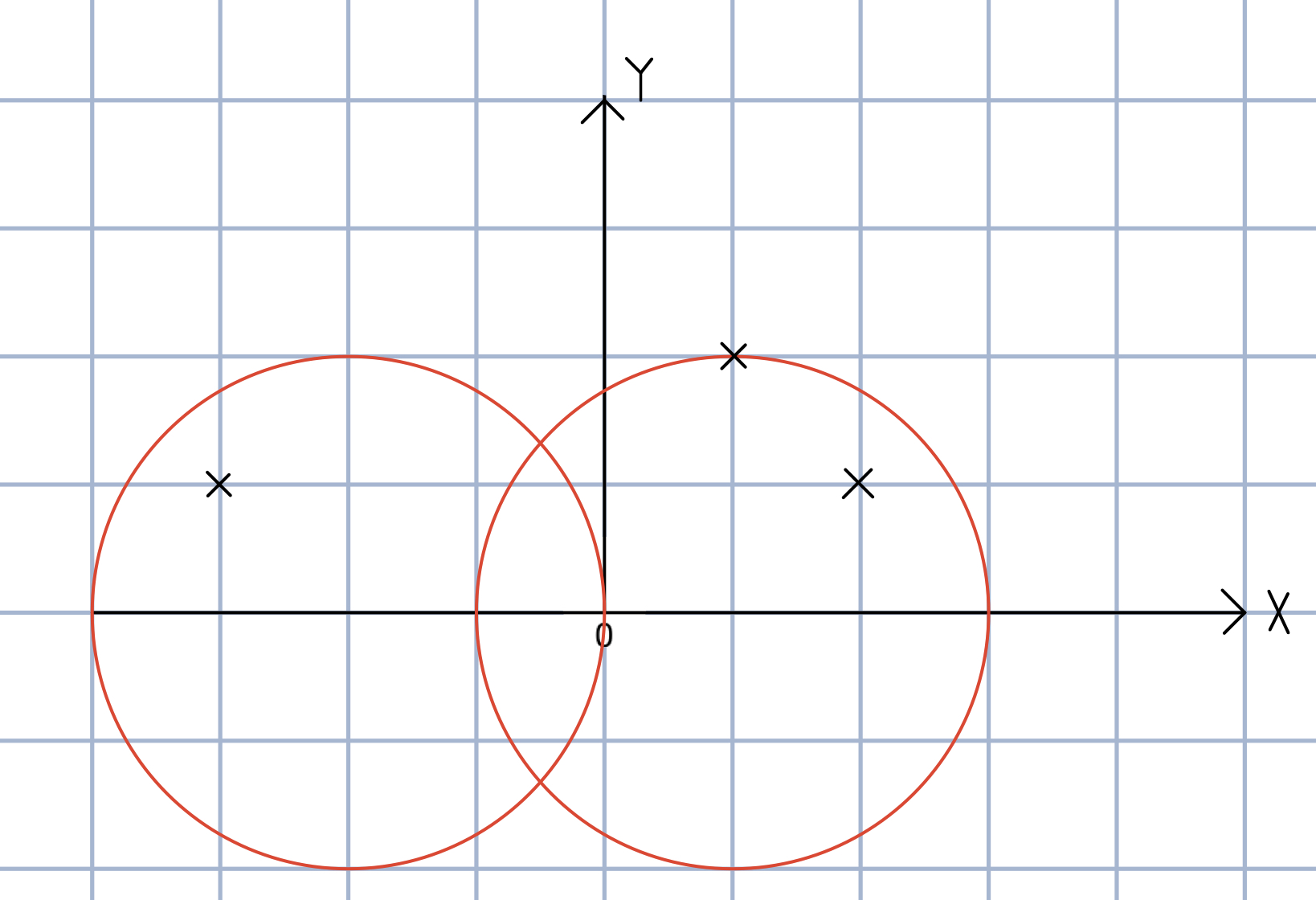## C、Guard the empire（贪心）

Hbb is a general and respected by the entire people of the Empire.
The wizard will launch an attack in the near future in an attempt to
destroy the empire. Hbb had knew the way the Wizards will attack this
time: they will summon N bone dragons on the broad flat ground outside
the wall and let the bone dragons spray a flame (the flame can only be
emitted once). If the wall is sprayed by flames, the wall will be
completely destroyed in an instant. To withstand the wizard’s attack,
Hbb feels very anxious, although he already knew where all the bone
dragons would be summoned. Coincidentally, scientists at the
Capital Laboratory have developed a new type of weapon. The striking
range of the weapon is a circle with the weapon as the center and a
radius of D. In other words, if the weapons are properly placed, the
bone dragons within the strike range will be destroyed. Weapons can
only be placed on the wall, but Hbb is too anxious at this time to
know how to place the weapon, so he tells you the position of the bone
dragon . Since the cost of the weapon is very expensive, Hbb gives you
a requirement: tell him what the minimum number of weapons to use in
order to destroy all bone dragons. If there is no way to destroy all
bone dragons, output -1.

case contains two integers and , where is the number of bone dragon
in the ground and is the distance of coverage of the weapon. Then is
followed by lines each containing two integers and , representing
the coordinate of the position of each bone dragon. Then a blank line
follows to separate the cases. The input is terminated by a line
containing pair of zeros 输出描述: For each test case output one line
consisting of the test case number followed by the minimal number of
weapon needed. “-1” installation means no solution for that case. 示例1

``````3 2
1 2
-3 1
2 1

1 2
0 2

0 0
``````
``````输出
Case 1: 2
Case 2: 1
``````Case1 is explained in the figure, the wall is regarded as the X axis, and the weapon can only be placed on the X axis. The red circle is the weapon strike range.

#### 题解如下

``````#include<iostream>
#include<cmath>
#include<algorithm>
#define l first
#define r second
#define Pff pair<double,double>			//这里的 pair 用的非常恰到好处，pair的两个值恰好存储区间的两个端点
using namespace std;

const int Len = 1005;
Pff p[Len];
int n; double d;

inline void cal(int &x,int &y,int &i)	//计算某个骨龙所对应的武器放置的区间
{
p[i].l = (double)x - sqrt(d * d - y * y);
p[i].r = (double)x + sqrt(d * d - y * y);
}

inline void work()
{
sort(p + 1, p + 1 + n);			//对各个武器区间进行排序
int ans = 1;
double pos_r = p.r;
for(int i = 2; i <= n; ++i)
{
if(p[i].l > pos_r)
{
++ ans;
pos_r = p[i].r;
}
else if(p[i].r < pos_r)		//⚠️这里的一步操作一定要理解
{
pos_r = p[i].r;
}
}
printf("%d\n",ans);
}

int main()
{
int case_ = 1;
while(~scanf("%d %lf", &n, &d) && n + d)
{

printf("Case %d: ",case_ ++);
int flag = 0,x,y;
for(int i = 1;i <= n;++ i)
{
scanf("%d %d",&x,&y);
if(y > d || flag)
{
flag = 1;     //在输入的时候不能够break ，否则就输不进去了
continue;
}
cal(x,y,i);
}
if(flag == 0)
work();
else
printf("-1\n");
}
return 0;
}
``````